# Chapter 1 - Particulate Solids¶

## Page 13 Example 1.1¶

In :
from __future__ import division
%matplotlib inline
from matplotlib.pyplot import plot,title,xlabel,ylabel,show
#Given size analysis of a powdered material
d=[1,101]##diameter of the powdered particles
x=[0,1]##mass fractions of the particles
plot(d,x)
title("size analysis of powder")
xlabel("particle size(um)")
ylabel("mass fraction(x)")
show()
#d=100*x+1# # from the given plot
#calculation of surface mean diameter
def surface_mean_diameter(x0,x1):
return ds

ds=surface_mean_diameter(0,1)##deduced surface mean diameter according to def.
print"\n The surface mean diameter is %.3f um"%(ds)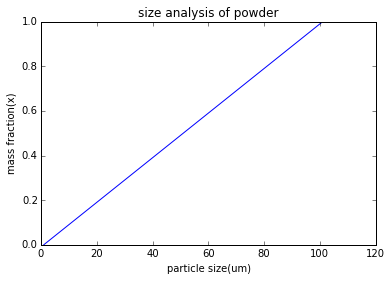The surface mean diameter is 21.668 um


## Page 14 Example 1.2¶

In :
from __future__ import division
%matplotlib inline
from matplotlib.pyplot import plot,title,xlabel,ylabel,show

#from given differential eq we get these functions
#particle number distribution for the size range 0-10um

#n=0.5*d**2#
#const of integration is0 since at n=0,d=0

#particle number distribution for the size range 10-100um
#n=83-(0.33*(10**(5))*d**(-3))
#c2=83,since at d=10um,n=50

#number distribution plot for the powdered material of size range 0-100um
def number_distribution(d):
if(d<=10):
n=0.5*d**2#
else:
n=83-(0.33*(10**(5))*d**(-3))#
return n
d=0#
n=[]
while(d<=100):
n.append(number_distribution(d))
d=d+1#
plot(range(0,101),n)
title("number_distribution_plot")
xlabel("diameter(um)")
ylabel("number distribution")
show()

ps=[0, 6.2, 9.0 ,10.0, 11.4, 12.1, 13.6 ,14.7 ,16.0 ,17.5 ,19.7 ,22.7, 25.5, 31.5 ,100]
def difference(i):
#ps=[0 6.2 9.0 10.0 11.4 12.1 13.6 14.7 16.0 17.5 19.7 22.7 25.5 31.5 10]#
#according to the given particle sizes particle sizes are in um
n1=number_distribution(ps[i])-number_distribution(ps[i-1])#
return n1
def average(i):
da= (ps[i]+ps[i-1])/2#
return da
tot_n1d12=0#
tot_n1d13=0#
i=1#
for i in range(1,15):
tot_n1d12=tot_n1d12+difference(i)*(average(i))**2
tot_n1d13=tot_n1d13+difference(i)*(average(i))**3

print"\n tot_n1d12 =%d \n tot_n1d13=%d"%(tot_n1d12,tot_n1d13)
def surface_area(j):
s=(difference(j)*(average(j))**2)/tot_n1d12
return s

j=0#

plot(0,0)
su=
for j in range(1,15):
su.append(su[j-1]+surface_area(j))
plot(ps[0:15],su)

title("surface area and mass distribution plot")
xlabel("diameter(um)")
ylabel("surface area or mass distribution")

#mass distribution plot
def mass_distribution(k):
x=(difference(k)*(average(k))**3)/tot_n1d13#
return x

ma=#
k=0#
plot(0,0)
for k in range(1,15):
ma.append(ma[k-1]+mass_distribution(k))
plot(ps[0:15],ma)
show()
#evaluating surface mean diameter
def surface_mean_diameter():
e=0#
for l in range(1,15):
n=(mass_distribution(l)/average(l))#
e=e+n#

d=1/e#
return d
print"\nthe surface mean diameter is: %.3f um"%(surface_mean_diameter())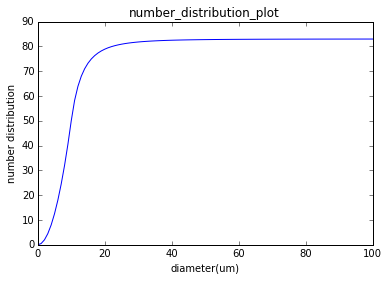tot_n1d12 =13513
tot_n1d13=423975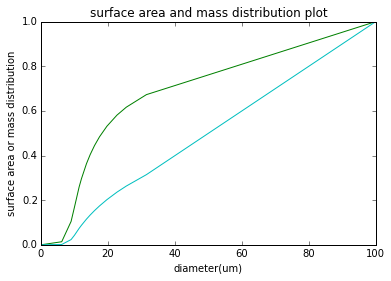the surface mean diameter is: 31.374 um


## Page 32 Example 1.3¶

In :
from __future__ import division
%matplotlib inline
from matplotlib.pyplot import plot,title,xlabel,ylabel,show
p=0.20##components analysed represents 20 percent of the mixture by mass
#for a completely unmixed system
so=p*(1-p)#
#for a completely random mixture :
n=100##Each of the sample removed contains 100 particles
sr=p*(1-p)/n#
s=[0.025, 0.006, 0.015, 0.018 ,0.019]
time_secs=[30, 60 ,90 ,120 ,150]
print"\n degree of mixing is :\n"
b=[]
def degree_of_mixing():
for i in range(0,5):
b.append((so-s[(i)])/(so-sr))
print "    %0.5f"%b[(i)]##b is the degree of mixing

return b#

plot(time_secs,degree_of_mixing())
title("degree of mixing curve")
xlabel("time_secs")
ylabel("degree_of_mixing")
show()
#plot of sample variance vs time(secs)
plot(time_secs,s)
title("sample variance curve")
xlabel("time_secs")
ylabel("sample variance")
show()
#from the graph the maxima is at 60 secs

 degree of mixing is :

0.89015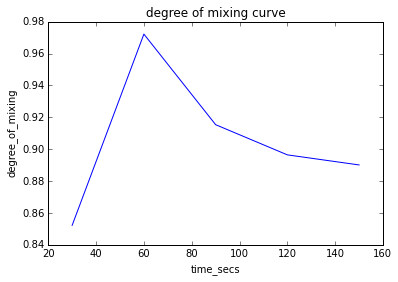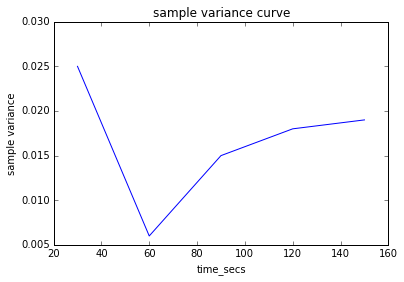## Page 39 Example 1.4¶

In :
from sympy import symbols,solve
#maximum size of the particle(mm)
d_max=0.065#
#minimum size of the particle(mm)
d_min=0.015#
#density of quartz(kg/m**3)
p_quartz=2650#
#density of galena (kg/m**3)
p_galena=7500#
#minimum density of the particle which will give this seperation
#When stoke's law is applied the required density is as given below
def stoke_required_density():
p=symbols('p')
d=solve((p-7500)-(p-2650)*(d_max/d_min)**2)
return d
d=stoke_required_density()#
print"\n required density is = %d kg/m**3"%(d)
#When Newton's law is applied then the required density is as given below
def newton_required_density():
r=symbols('r')
e=solve((r-7500)-(r-2650)*(d_max/d_min))
return e
e=newton_required_density()#
print"\nrequired density is by newton law =%d kg/m**3"%(e)

 required density is = 2377 kg/m**3

required density is by newton law =1195 kg/m**3


## Page 71 Example 1.5¶

In :
#efficiency of the collector for different size ranges
efficiency_1=45##in percentage for the size range of 0-5um
efficiency_2=80##in percentage for the size range of 5-10um
efficiency_3=96##in percentage for the size range greater than 10um

#mass percent of the ndust for various size range
mass_1=50# #in percentage for the size range of 0-5um
mass_2=30# #in percetage for the size range of 5-10um
mass_3=20# #in percentage for the size range greater than 10um
# on the basis of 100kg dust
mass_retained_1=0.45*50##mass retained(kg) in the size range of 0-5um
mass_retained_2=0.80*30##mass retained(kg) in the size range of 5-10um
mass_retained_3=0.96*20##mass retained(kg) in the size range greater than10um
overall_efficiency=0.45*50+0.80*30+0.96*20#
print"\n the overall efficiency is = %.1f"%(overall_efficiency)#

 the overall efficiency is = 65.7


## Page 71 Example 1.6¶

In :
from __future__ import division
#To calculate mass flow of the dust emitted
mass_1=10##in percentage in the size range of 0-5um
mass_2=15##in percentage in the size range of 5-10um
mass_3=35##in percentage in the size range of 10-20um
mass_4=20##in percentage in the size range of 20-40um
mass_5=10##in percentage in the size range of 40-80um
mass_6=10##in percentage in the size range of 80-160um
efficiecny_1=20##in percentage in the size range of 0-5um
efficiency_2=40##in percentage in the size range of 5-10um
efficiency_3=80##in percentage in the size range of 10-20um
efficiency_4=90##in percentage in the size range of 20-40um
efficiency_5=95##in percentage in the size range of 40-80um
efficiency_6=100##in percentage in the size range of 80-160um
dust_burden=18##in g/m**3 at the entrance
#taking 1m**3 as the basis of calculation
total_mass_retained=18*(0.1*0.20+0.15*0.40+0.35*0.80+0.2*0.9+0.1*0.95+0.1*1)#
print"\ntotal mass retained = %f g"%(total_mass_retained)#
total_efficiency=(total_mass_retained/18)*100#
print"\ntotal efficiency is = %f"%(total_efficiency)#
total_mass_emitted=18-total_mass_retained#
print"total mass emitted is:%f g"%(total_mass_emitted)#
t=18*(0.1*0.80+0.15*0.60+0.35*0.20)#
print"\ntotal mass emitted less than 20 um is %fg"%(t)
e=t*100/total_mass_emitted#
print"\nThe efficiency of particles emitted is %f"%(e)#
#gas flow is 0.3m**3/sec
f=0.3*total_mass_emitted#
print"\nmass flow rate is:%f kg/sec"%(f)#


total mass retained = 13.230000 g

total efficiency is = 73.500000
total mass emitted is:4.770000 g

total mass emitted less than 20 um is 4.320000g

The efficiency of particles emitted is 90.566038

mass flow rate is:1.431000 kg/sec


## Page 79 Example 1.7¶

In :
from math import pi
from __future__ import division
Ai=(pi/4)*(0.075)**2##cross sectional area at the gas inlet in m**2
do=0.075##gas outlet diameter in m
p=1.3##gas density in kg/m**3
Z=1.2##height of the seperator in m
dt=0.3##seperator diameter in m
v=1.5##gas entry velocity in m/sec
G=(Ai*v*p)##mass flow rate of the gas in kg/sec
print"\n cross sectional area at the gas inlet is %f m**2"%(Ai)#
print"\ngas outlet diameter is %f m"%(do)#
print"\ngas density is %f kg/m**3"%(p)#
print"\nheight of the seperator is %f m"%(Z)#
print"\nseperator diameter is%f m"%(dt)#
print"\nmass flow rate of the gas is %f kg/sec"%(G)
def terminal_vel():
u=0.2*(Ai)**2*(do)*p*9.8/(pi*Z*(dt)*G)##velocity is in m/sec
return u

u=terminal_vel()#
print"\nthe terminal velocity of the smallest particle retained by the seperator =%fm/sec"%(u)#
def particle_diameter(u):
u=terminal_vel()#
n=0.018*10**(-3)##viscosity in mNs/m**2
ps=2700##density of the particle in kg/m**3
d=((u*18*n)/(9.8*(ps-p)))**(0.5)##particle size in um
return d
u=terminal_vel()#
d=particle_diameter(u)#
do=d*10**6#
print"\n particle diameter by the stoke law is %fum"%(do)

 cross sectional area at the gas inlet is 0.004418 m**2

gas outlet diameter is 0.075000 m

gas density is 1.300000 kg/m**3

height of the seperator is 1.200000 m

seperator diameter is0.300000 m

mass flow rate of the gas is 0.008615 kg/sec

the terminal velocity of the smallest particle retained by the seperator =0.000383m/sec

particle diameter by the stoke law is 2.165585um